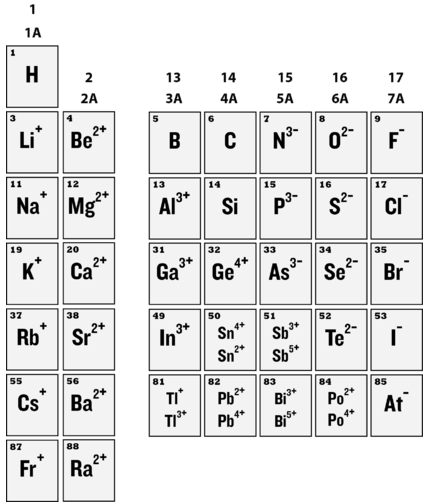# 8.4: Anion Formation

$$\newcommand{\vecs}{\overset { \rightharpoonup} {\mathbf{#1}} }$$ $$\newcommand{\vecd}{\overset{-\!-\!\rightharpoonup}{\vphantom{a}\smash {#1}}}$$$$\newcommand{\id}{\mathrm{id}}$$ $$\newcommand{\Span}{\mathrm{span}}$$ $$\newcommand{\kernel}{\mathrm{null}\,}$$ $$\newcommand{\range}{\mathrm{range}\,}$$ $$\newcommand{\RealPart}{\mathrm{Re}}$$ $$\newcommand{\ImaginaryPart}{\mathrm{Im}}$$ $$\newcommand{\Argument}{\mathrm{Arg}}$$ $$\newcommand{\norm}{\| #1 \|}$$ $$\newcommand{\inner}{\langle #1, #2 \rangle}$$ $$\newcommand{\Span}{\mathrm{span}}$$ $$\newcommand{\id}{\mathrm{id}}$$ $$\newcommand{\Span}{\mathrm{span}}$$ $$\newcommand{\kernel}{\mathrm{null}\,}$$ $$\newcommand{\range}{\mathrm{range}\,}$$ $$\newcommand{\RealPart}{\mathrm{Re}}$$ $$\newcommand{\ImaginaryPart}{\mathrm{Im}}$$ $$\newcommand{\Argument}{\mathrm{Arg}}$$ $$\newcommand{\norm}{\| #1 \|}$$ $$\newcommand{\inner}{\langle #1, #2 \rangle}$$ $$\newcommand{\Span}{\mathrm{span}}$$$$\newcommand{\AA}{\unicode[.8,0]{x212B}}$$Figure $$\PageIndex{1}$$: A pressed glass open salt dish made in the early 1830s. (Credit: User:Daderot/Wikimedia Commons; Source: http://commons.wikimedia.org/wiki/File:DAR_glass_-_IMG_8708.JPG(opens in new window); License: Public Domain)

### How do you make chlorine safe to eat?

How do you transform a deadly gas into something you can sprinkle on your eggs and eat for breakfast? Chlorine in its free form is very dangerous if you breathe the fumes or come in contact with the gas. However, in reaction with sodium, sodium chloride forms as the sodium atom gives up an electron to chlorine, which accepts the electron to form the chloride anion.

## Anions

Anions are the negative ions formed from the gain of one or more electrons. When nonmetal atoms gain electrons, they often do so until their outermost principal energy level achieves an octet. This process is illustrated below for the elements fluorine, oxygen, and nitrogen:

$\begin{array}{lcl} \ce{F} + \ce{e^-} & \rightarrow & \ce{F^-} \\ 1s^2 \: 2s^2 \: 2p^5 & & 1s^2 \: 2s^2 \: 2p^6 \: \text{(octet)} \end{array}\nonumber$

$\begin{array}{lcl} \ce{O} + 2 \ce{e^-} & \rightarrow & \ce{O^{2-}} \\ 1s^2 \: 2s^2 \: 2p^4 & & 1s^2 \: 2s^2 \: 2p^6 \: \text{(octet)} \end{array}\nonumber$

$\begin{array}{lcl} \ce{N} + 3 \ce{e^-} & \rightarrow & \ce{N^{3-}} \\ 1s^2 \: 2s^2 \: 2p^3 & & 1s^2 \: 2s^2 \: 2p^6 \: \text{(octet)} \end{array}\nonumber$

All of these anions are isoelectronic with each other and with neon. They are also isoelectronic with the three cations from the previous section. Under typical conditions, three electrons are the maximum that will be gained in the formation of anions.

Outer electron configurations are constant within a group, so this pattern of ion formation repeats itself for Periods 3, 4, and following (see below).Figure $$\PageIndex{2}$$: Ion charges. (Credit: Christopher Auyeung; Source: CK-12 Foundation; License: CC BY-NC 3.0(opens in new window))

It is important not to misinterpret the concept of being isoelectronic. A sodium ion is very different from a neon atom, because the nuclei of the two contain different numbers of protons. One is an essential ion that is a part of table salt, while the other is an unreactive gas that is a very small part of the atmosphere. Likewise, sodium ions are very different than magnesium ions, fluoride ions, and all the other members of this isoelectronic series $$\left( \ce{N^{3-}}, \: \ce{O^{2-}}, \: \ce{F^-}, \: \ce{Ne}, \: \ce{Na^+}, \: \ce{Mg^{2+}}, \: \ce{Al^{3+}} \right)$$.Figure $$\PageIndex{3}$$: Neon gas (A) and sodium chloride crystals (B). Neon atoms and sodium ions are isoelectronic. Neon is a colorless and unreactive gas that glows a distinctive red-orange color in a gas discharge tube. Sodium ions are most commonly found in crystals of sodium chloride—ordinary table salt. (Credit: (A) Andy Wright (Flickr: rightee); (B) Kevin Dooley;User:Bugman/Wikipedia; Source: (A) http://www.flickr.com/photos/rightee/4356950/(opens in new window); (B) http://www.flickr.com/photos/pagedooley/2769134850/(opens in new window); http://commons.wikimedia.org/wiki/File:Ion_exchange_resin_beads.jpg(opens in new window); License: CC by 2.0(opens in new window))

## Summary

• Anions are negative ions formed by accepting electrons.
• The outermost principal energy level of an anion is usually an octet.

## Review

1. What is an anion?
2. Write the electronic configurations for the chlorine atom and the chloride anion.
3. What does isoelectronic mean?

This page titled 8.4: Anion Formation is shared under a CK-12 license and was authored, remixed, and/or curated by CK-12 Foundation via source content that was edited to the style and standards of the LibreTexts platform; a detailed edit history is available upon request.